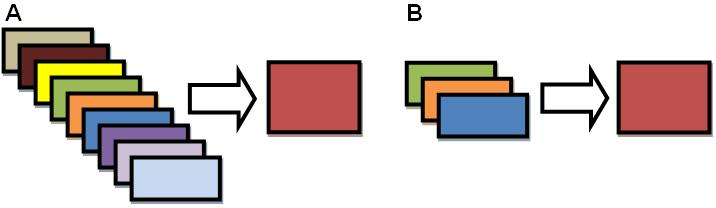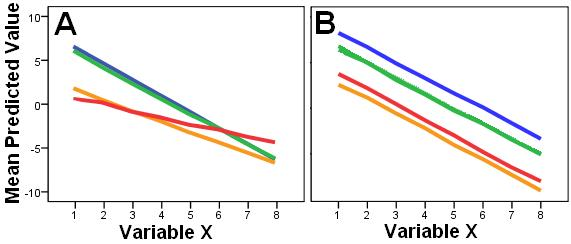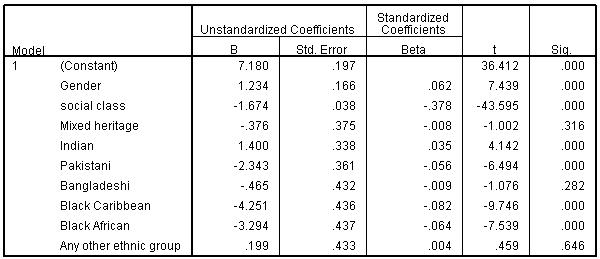# Using Statistical Regression Methods in Education Research# Multiple Linear Regression Module Quiz

Your total score is :0 / 15

Welcome to the Multiple Linear Regression Module quiz where we challenge your growing knowledge with a fresh set of 15 fiendish questions. Click VIEW FEEDBACK when you’re ready to see the answers. When you want to move on just click EXIT QUIZ. Enjoy!
1. You want to construct a multiple linear regression model that predicts a pupil’s score on a reading test (%) based on a number of important factors. Sort the following variables according to how you will use them in your model:
 Factors Continuous Explanatory Dichotomous Explanatory Dummy Explanatory Outcome Pupil Gender Geographic region of school (6 categories) Pupil Ethnicity (8 categories) Income of family (£) Hours per week spent reading Reading Test Score (%) Free school meal eligibility (yes/no)

2. Imagine that each of the squares below represents a variable. The two diagrams represent models which both explain 75% of the variance in the outcome. Which looks to be the more parsimonious model?4. A multiple linear regression with two Explanatory variables is carried out, explaining 70% of the total variance in the outcome. Variable A uniquely accounts for 30% of the total variance and Variable B for 25% of the total variance. What accounts for the remaining 15% of variance which has been explained?

6. Graphs A and B are P-P plots and can be used to ascertain whether or not the residuals for your regression analysis are normally distributed.Which of the two P-P plots might give you cause for concern?

7. If a case has a high residual statistic, what does this suggest about the accuracy of the model for predicting that case’s score on the outcome measure?

8. Is it possible to talk sensibly about a main effect for a variable if that variable is involved in an interaction with another explanatory variable?

9. Two regression models are completed, both of which include interaction terms. Which of these two line graphs suggests an interaction effect is occurring between variable X (x-axis) and Variable Z (represented by the different coloured lines)? The mean predicted score on the outcome varible is on the Y-axis.10. Drag the correct statements into the relevant box for each term
 Statements Multicollinearity Homoscedasticity Outlier Examining a scatterplot of residuals against predicted outcome can help you identify this issue The variance of the residuals is the same at each level of the predictor variable(s) A single case which has a disproportionately strong influence over your regression model The 'Cook's distance' statistic can be used to look for this issue The 'variance inflation factor' (VIF) can be used to identify this issue Two or more predictor variables are highly correlated with one another

11. A multiple linear regression using standardised age 14 exam score as an outcome variable was carried out. The reference category for the ethnicity variables is White-British. Males were coded as ‘0’ and females as ‘1’. Social class is an ordinal variable with 0 representing the most affluent homes and 8 the least affluent homes).Which of the following two statements are true about the coefficients table:

12. In the regression table for the question above which of the ethnic categories do not differ from the White British category to a statistically significant level?

13. Interaction terms were added to the regression model from the question above. Which of the following statements about the regression coefficients table shown below are true?14. We can be 95% certain that the first coefficient in the list below would not have a value of less than -7.299 if we repeated the regression analysis with another sample randomly drawn from the same population15. A regression analysis was completed in two blocks, the second of which added interaction terms to the explanatory variables included in the first model. Did the addition of the interaction terms improve the amount of variance explained to a statistically significant degree?Privacy statement
This quiz is anonymous. No data which personally identifies you is collected on the quiz, and the data you provide is used solely to help us improve the delivery of our courses.
Page contact: Feedback to ReStore team Last revised: Mon 13 Sep 2010